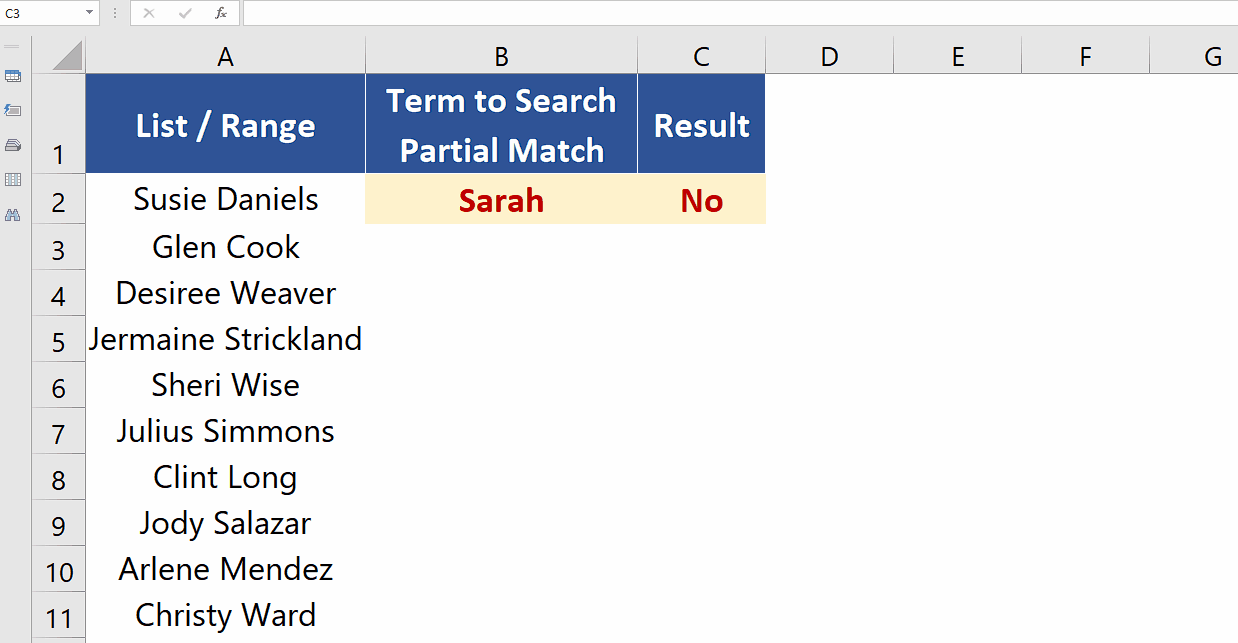# How to check if a value exists in a range in Excel

In this tutorial we’ll learn how to check if a specific name exists in a list of names in Excel. Let’s look at the dataset given above in which we have a list of names and we will search for a name by two ways, i.e., exact match and partial match. Let’s follow the steps explained below to find out the ways to do it.Microsoft Excel is famous for numeric calculations based upon mathematical and statistical functions but at the same time it provides us functions to perform logical operations as well. Sometimes, you might want to check if a specific value exists in a range to perform some action based on the result of the check. For example, you might want to display a message or highlight a cell if the value exists within a certain data range.

### Step 1 – Use COUNTIF with IF to find exact match– We can use a combination of COUNTIF and IF functions to create a simple formula which will count the number of occurrences of a specific value in the range and if the value is found once or more than once then YES will be displayed, otherwise a NO will be displayed. The formula will be as follows,
=IF(COUNTIF(A2:A11,B2)>0, “YES”, “NO”)

In our case A2:A11 is the range that contains the list of names and B2 is the cell which contains the value to be searched as shown above.

### Step 2 – Use COUNTIF with wildcard and IF to find partial matches– We can use a combination of COUNTIF and IF functions to create a simple formula which will count the number of occurrences of a specific value in the range and if the value is found once or more than once then YES will be displayed, otherwise a NO will be displayed. The formula will be as follows,
=IF(COUNTIF(A2:A11,”*”&B2&”*”)>0,”Yes”,”No”)

In our case A2:A11 is the range that contains the list of names and B2 is the cell which contains the value to be searched as shown below. This formula uses wildcard * before and after the text to be searched to find the partial matches as shown below.